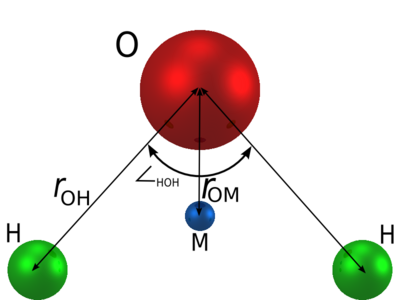TIP3P model of water

(Redirected from TIP3P)

The TIP3P model of water .

Parameters

The TIP3P model consists of a Lennard-Jones site for the oxygen atom, and two charge sites.$r_{\mathrm {OH}}$ (Å)$\angle$HOH , deg$\sigma$ (Å)$\epsilon$ (kJ/mol) q(O) (e) q(H) (e) q(M) (e)$r_{\mathrm {OM}}$ (Å) 0.9572 104.52 3.15066 0.63627 -0.834 0.417 0 0

Surface tension

The surface tension has been studied for the TIP3P model by Vega and Miguel 

Shear viscosity

The shear viscosity for the TIP3P model is 0.321 mPa.s at 298 K and 1 bar  (experimental value 0.896 mPa.s ).